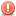A Flash Developer Resource Site

1. ##Multiple HitTests Help! Please!

Hi there I've been reading up and looking at examples of how to handle multiple hittests. So far in my example I have failed.
PHP Code:
``` ///Variablesvar Xdist:Number = 0;var Ydist:Number = 0;var partAng:Number = 0;var bulletXvel:Number = 0;var bulletYvel:Number = 0;var bulletAcc:Number = 2;var bulletDec:Number = 0.98;var firePower:Number = 12;///Arrays//Stores Bulletsvar particleArray:Array = [ ];//Stores Atomsvar atomArray:Array = [ ];//Stores the individual Atom's X'n'Y Positionvar atomPosX:Array = [600,200];var atomPosY:Array = [150,150];//Creates the Atoms using MC from the library and X'n'Y Pos Arrays, then stores the created in an array.for(var i=0; i<atomPosX.length; i++){    var nuke = new atom();        nuke.x = atomPosX[i];    nuke.y = atomPosY[i];        atomArray.push(nuke);    addChild(nuke);}stage.addEventListener(Event.ENTER_FRAME, eachFrame);function eachFrame(event:Event):void {    //Trig for barrel position    Xdist = (turret.x - mouseX);    Ydist = (mouseY - turret.y);    turret.barrel.rotation = -((Math.atan2(Ydist,Xdist)) * (180/Math.PI));    //Move Particles    for(var i=0; i<particleArray.length; i++) {        //Temporary name storing var        var part = particleArray[i];        //Moves the bullet by its individual velocities        part.x += part.xmov;        part.y += part.ymov;        //Removes bullets when they hit the bounds of the stage        if(part.x > 850 || part.x < -50 || part.y < -50 || part.y > 650) {            removeChild(part);            particleArray.splice(i, 1);        }    }}stage.addEventListener(MouseEvent.MOUSE_DOWN, fireParticle);function fireParticle(event:MouseEvent):void {    var part = new electron();        partAng = turret.barrel.rotation;    //Intial X'n'Y Position of the bullet    part.x = turret.x+(70* Math.cos((turret.barrel.rotation+180)* Math.PI/180));    part.y = turret.y+(70* Math.sin((turret.barrel.rotation+180)* Math.PI/180));    //Initial bullet velocities.    part.xmov = -Math.cos(partAng*Math.PI/180)* firePower;    part.ymov = -Math.sin(partAng*Math.PI/180)* firePower;    //Storing bullet in the array;    particleArray.push(part);    addChild(part);}  ```
Basically I want to be able to check for a hitTest against all the bullets on stage against all atoms created. I attempted to do this with two for loops and it didn't work can anyone help me with this specific problem. Project folder attached.Reply With Quote

2. one problem I see off the bat is here:

PHP Code:
```   for(var i=0; i<particleArray.length; i++) {        //Temporary name storing var        var part = particleArray[i];        //Moves the bullet by its individual velocities        part.x += part.xmov;        part.y += part.ymov;        //Removes bullets when they hit the bounds of the stage        if(part.x > 850 || part.x < -50 || part.y < -50 || part.y > 650) {            removeChild(part);            particleArray.splice(i, 1);  // <<<<<< RIGHT HERE        }    }  ```
your for loop is going though the length of the particleArray, but if you splice off part of the array it gets shorter, so it skips the next bullet

there are a few ways to get around this. here is one way:
PHP Code:
```   for(var i=0; i<particleArray.length; i++) {        //Temporary name storing var        var part = particleArray[i];        //Moves the bullet by its individual velocities        part.x += part.xmov;        part.y += part.ymov;        //Removes bullets when they hit the bounds of the stage        if(part.x > 850 || part.x < -50 || part.y < -50 || part.y > 650) {            removeChild(part);            particleArray.splice(i, 1);            i--;  // we subtract on from i, to keep from skipping an element.        }    }  ```
let us know if there are other problems after doing this, since its the first thing I found. = DReply With Quote

####Posting Permissions

• You may not post new threads
• You may not post replies
• You may not post attachments
• You may not edit your posts
•

 » Home » Movies » Tutorials » Submissions » Board » Links » Reviews » Feedback » Gallery » Fonts » The Lounge » Sound Loops » Sound FX » About FK » Sitemap